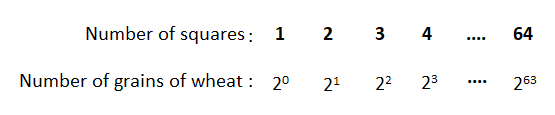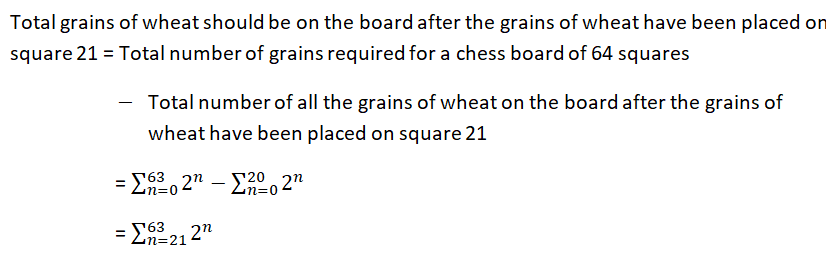# A King in ancient times agreed to reward the inventor of chess with one grain of wheat on the first of the 64 squares of a chess board. On the second square the King would place two grains of wheat, on the third square, four grains of wheat, and on the fourth square eight grains of wheat. If the amount of wheat is doubled in this way on each of the remaining squares, how many grains of wheat should be placed on square 2121 ? Also find the total number of grains of wheat on the board at this time and their total weight in pounds. (Assume that each grain of wheat weighs 1/7000 pound.)How many grains of wheat should be placed on square 2121 ?nothinggrainsHow many total grains of wheat should be on the board after the the grains of wheat have been placed on square 2121 ?nothinggrainsWhat is the total weight of all the grains of wheat on the board after the grains of wheat have been placed on square 2121 ?nothingpounds(Round to the nearest tenth as needed.)

Question
2 views

A King in ancient times agreed to reward the inventor of chess with one grain of wheat on the first of the 64 squares of a chess board. On the second square the King would place two grains of wheat, on the third square, four grains of wheat, and on the fourth square eight grains of wheat. If the amount of wheat is doubled in this way on each of the remaining squares, how many grains of wheat should be placed on square
21
21 ? Also find the total number of grains of wheat on the board at this time and their total weight in pounds. (Assume that each grain of wheat weighs 1/7000 pound.)
How many grains of wheat should be placed on square
21
21 ?

nothing
grains
How many total grains of wheat should be on the board after the the grains of wheat have been placed on square
21
21 ?

nothing
grains
What is the total weight of all the grains of wheat on the board after the grains of wheat have been placed on square
21
21 ?

nothing
pounds
(Round to the nearest tenth as needed.)

check_circle

Step 1

A King in ancient times agreed to reward the inventor of chess with one grain of wheat on the first of the 64 squares of a chess board. On the second square the King would place two grains of wheat, on the third square he would place four grains of wheat, and on the fourth square he would place eight grains of wheat.

If the amount of wheat is doubled in this way on each of the remaining squares, then the corresponding number of grains of wheat and number of squares will be as follows.Step 2

So, number of grains of wheat should be placed on square 21 is 220 = 1048576.

Assume that each grain of wheat weighs 1/7000 pound.

And their total weight in pounds will be, (1/7000)*1048576 =149.79657 ≈ 149.79 pound.

Step 3

Now, we need to find how many total grains of wheat should be on the board after the grains of wheat have been placed on square 21.The above number is a very big number.

...

### Want to see the full answer?

See Solution

#### Want to see this answer and more?

Solutions are written by subject experts who are available 24/7. Questions are typically answered within 1 hour.*

See Solution
*Response times may vary by subject and question.
Tagged in